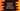# JavaScript program to add padding to the start of a string## How to add padding to the start of a string in JavaScript using padStart:

JavaScript string has one method called padStart that can be used to pad a string with another string. We can provide a length for the final string and it will create a new string by padding the original string with another string.

In this post, we will learn how to use this method with examples.

### Definition of padStart:

This method is defined as like below:

``padStart(length, str)``

Here,

• length is the final length of the string. If the value of this is smaller than the string length, then it will return the string without making any change.
• str is an optional value. This is used to pad the current string. If it is large in size, it is truncated from the end. By default, it uses space.

### Return value of padStart:

This method will return the final string of length length with str added from the start.

### Example of padStart:

Let’s take different examples of padStart:

``````const givenStr = "Hello";

It will give the below output:

``````     Hello
*****Hello
Hello
12312Hello
12345Hello``````
• For example 1, it added 5 blank spaces at the start of the string givenStr to make it length 10.
• For example 2, it added 5 * at the start of the string to make it length 10.
• For example 3, it didn’t make any change because 2 is smaller than the length of givenStr.
• For example 4, it added 123 and repeated it till the length become 10.
• For the last example, it added the string upto 5, and stops as it become 10.

If you provide a negative number, it will not make any change to the string. For example:

``````const givenStr = "Hello";

It will print:

``Hello``

Since, the negative number is always smaller than the length of the string, padStart will always return the string.

If you want to use padStart with a number, you need to convert the number to string. We can use the toString() method to convert a number to string. It can then use the padStart method on the string returned by toString.

Let’s take a look at the below program:

``````const givenNumber = 544321;

It will add four * to the front of givenNumber.

``****544321``

Similarly, we can use it with slice to get one part of a string and add characters to the front.

``````const givenNumber = 8989989890909999;

``************9999``